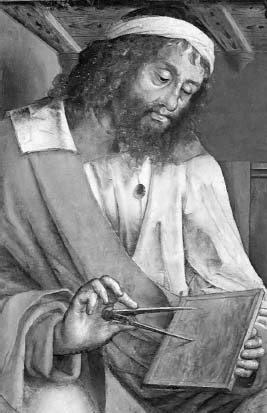NextPrevious

# What were Archimedes’s greatest contributions to mathematics?

Historians consider Archimedes (c. 287-212 B.C.E., Hellenic) to be one of the greatest Greek mathematicians of the classic era. Known for his discovery of the hydrostatic principle, he also excelled in the mechanics of simple machines; computed close limits on the value of “pi” by comparing polygons inscribed in and circumscribed about a circle; worked out the formula to calculate the volume of a sphere and cylinder; and expanded on Eudoxus’ method of exhaustion that would eventually lead to integral calculus. He also created a way of expressing any natural number, no matter how large; this was something that was not possible with Greek numerals. (For more information about Archimedes, see “Mathematical Analysis” and “Geometry and Trigonometry.”)The Greek mathematician Euclid contributed to the development of arithmetic and the geometric theory of quadratic equations.

Close

This is a web preview of the "The Handy Math Answer Book" app. Many features only work on your mobile device. If you like what you see, we hope you will consider buying. Get the App